# Improve overfitting by adjusting hyperparameters with no code - LightGBM

PUBLISHED
2021.04.04 (Sun)
CATEGORY
Tutorials
TOPIC
Model Performance Hyperparameters
18分39秒

The performance of a model is not only affected by the data, but also by the hyperparameters.

For complex models such as XGBoost, LightGBM, CatBoost, etc., the hyperparameters need to be adjusted correctly in order to take full advantage of their performance.
Nowadays, with the advent of optimization tools such as Optuna and Hyperopt, which are also supported by VARISTA, and AutoML, it is possible to perform automatic parameter tuning.

[Hyperparameter Optimization and Experiment Management with VARISTA Optuna Edition](/en/blog/hyperparameter-optimization-with-optuna-and-experiments-with-varista/ "Hyperparameter Optimization and Experiment Management with VARISTA Hyperparameter-optimization-with-optuna-and-experiments-with-varista/ "Hyperparameter Optimization and Experiment Management with Optuna")

However, in some cases, the model generated by AutoML or other software does not fully explore the parameters, and the model can be even better if the data scientist tweaks it manually.

In this article, I will explain how to tune hyperparameters in VARISTA using an overtrained model as an example.

The dataset we will use contains 7000 instances, 21 explanatory variables, and an objective variable.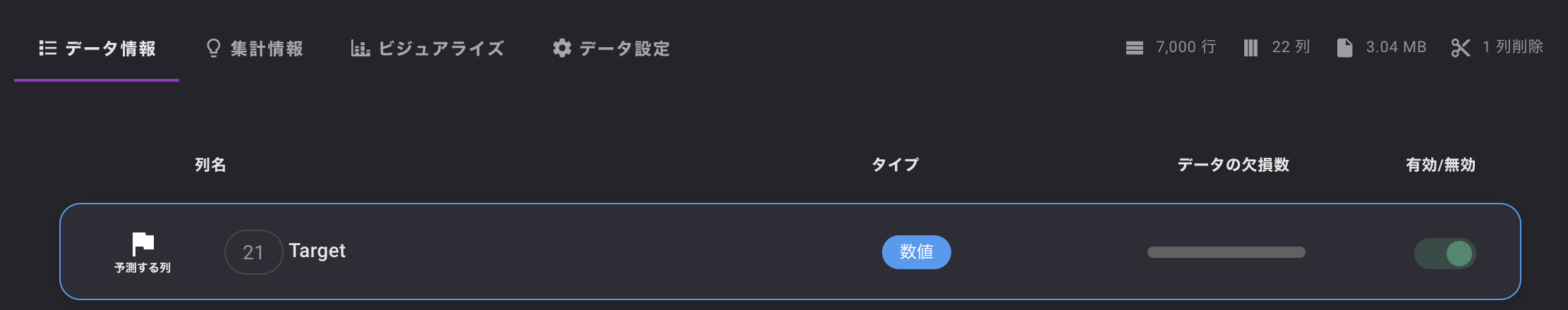Since the target is numeric, this is a regression task.

First, we will change the default template provided in VARISTA from XGBoost to LightGBM and train with the hyperparameters as they are.

The training takes about 20 seconds, so let's check the results.
There are multiple metrics displayed, but we will proceed with RMSE as the standard.
Shown below are the RMSE during training and validation when cross-validating the teacher data with Fold=3.

• train_neg_root_mean_squared_error: RMSE during training
• test_neg_root_mean_squared_error: RMSE during validation

VARISTA uses scikit-learn's cross-validation, so the RMSE value is negative.
3.3. Metrics and scoring: quantifying the quality of predictions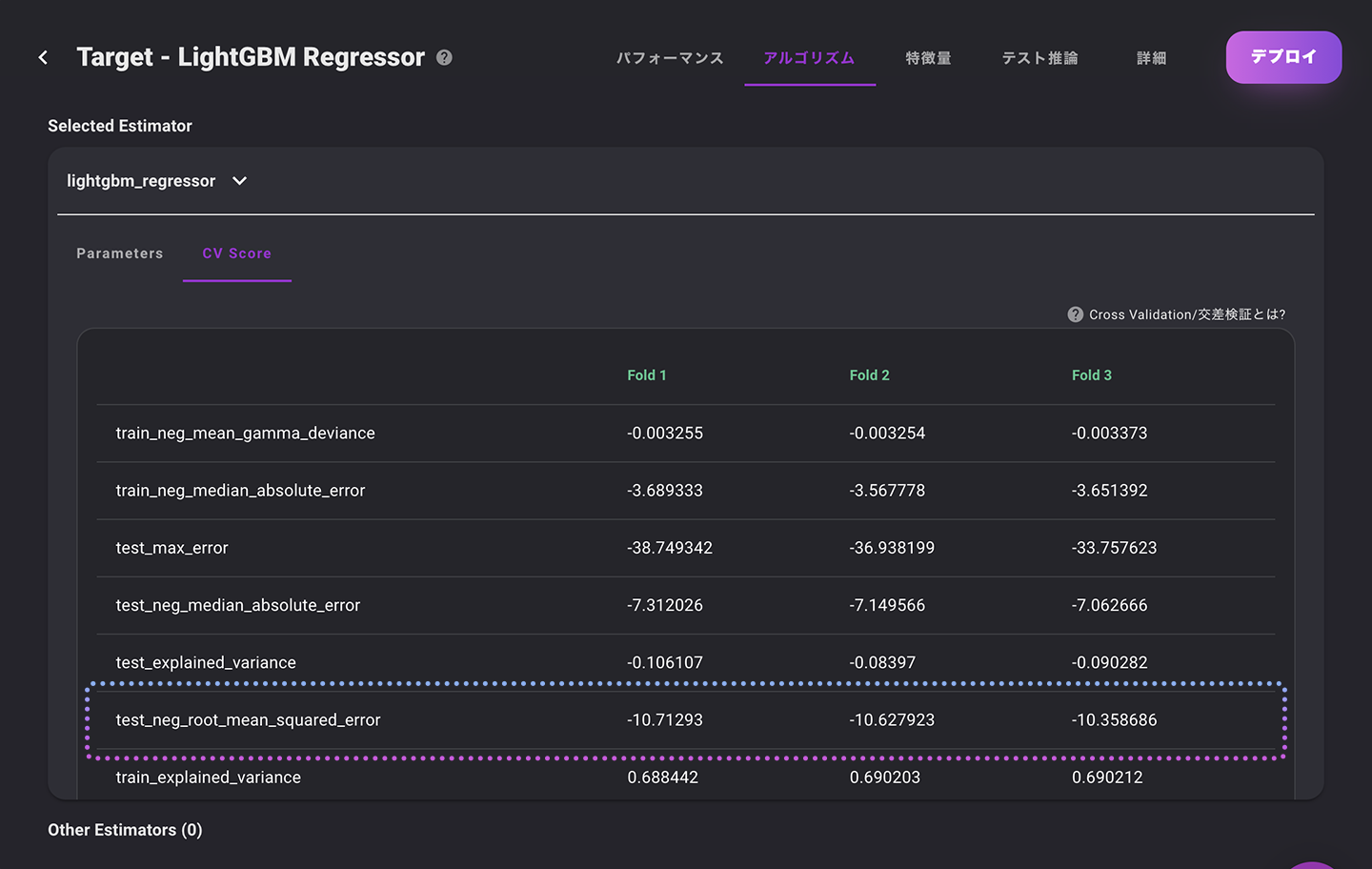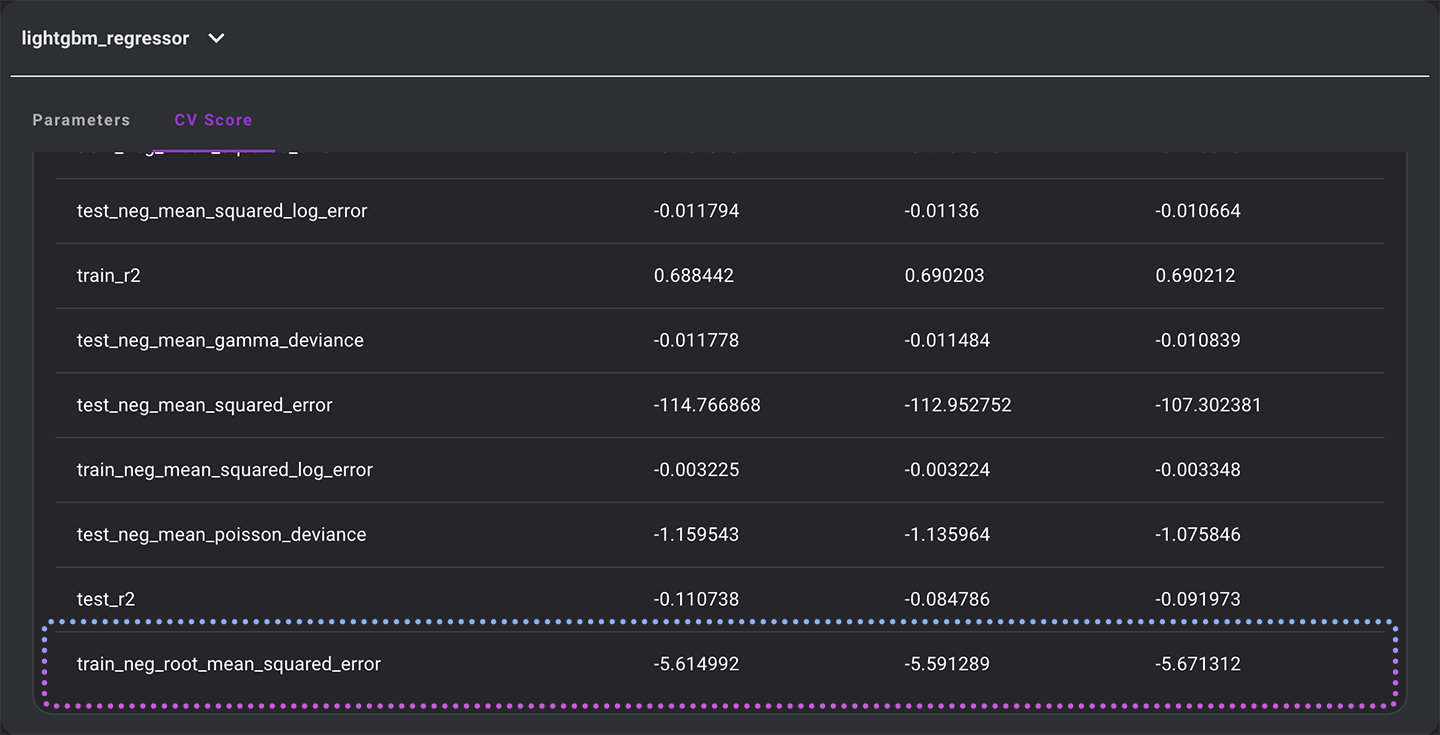The metrics we will focus on are extracted below for easy comparison.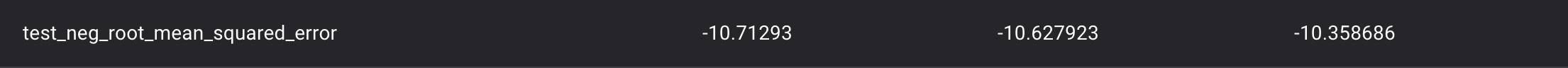The RMSE between training and validation is about -5, so the resulting model has been fitted to the training data. In other words, it is in a state of overtraining.

We will now improve the performance of the model by adjusting the hyperparameters from this state.

First, let's try to reduce overfitting by changing min_data_in_leaf.
We will adjust the number of data in each leaf to prevent the model from overfitting.
In this case, we will change this value to 100 and try to train it.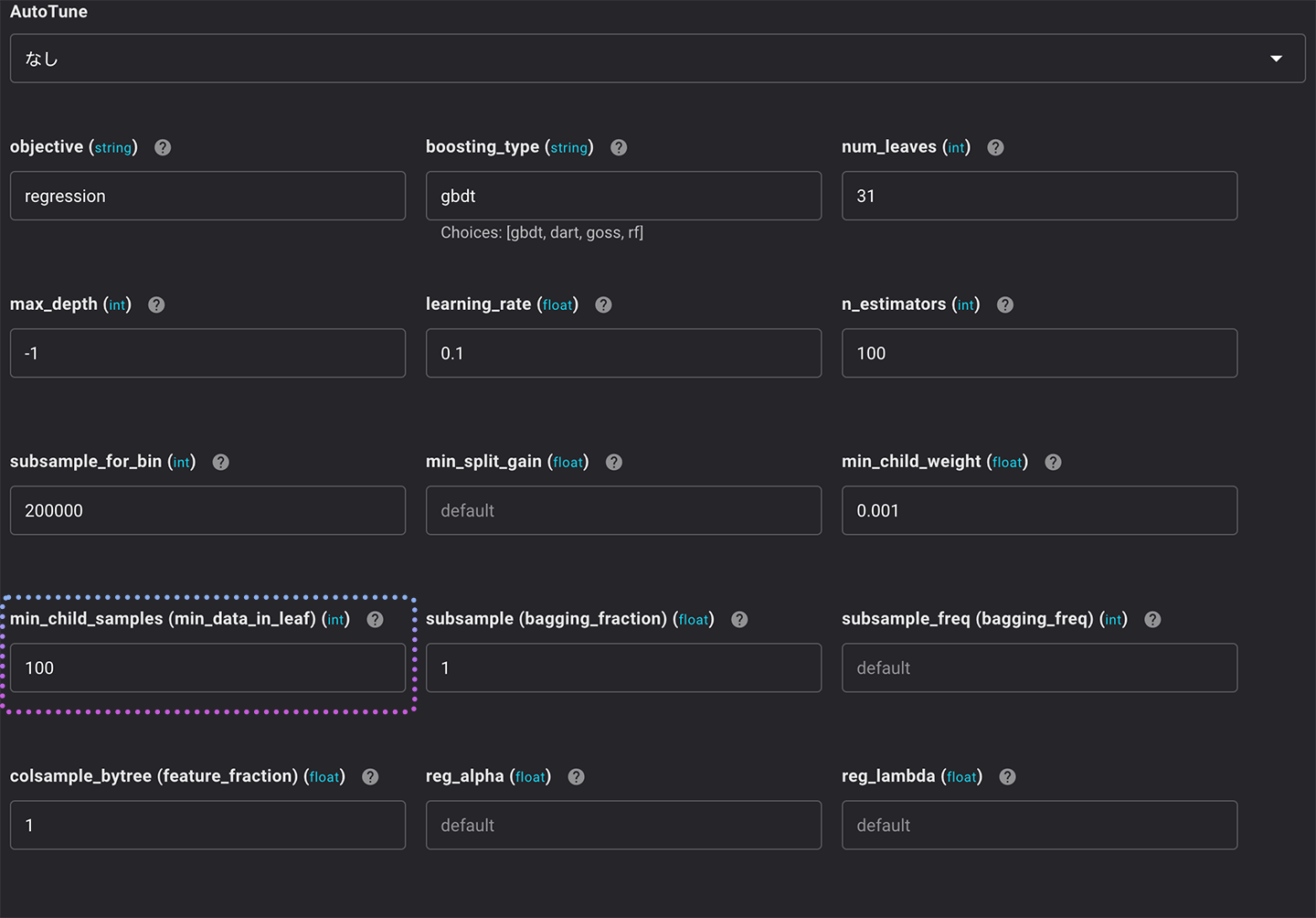Let's check the resulting model in this state.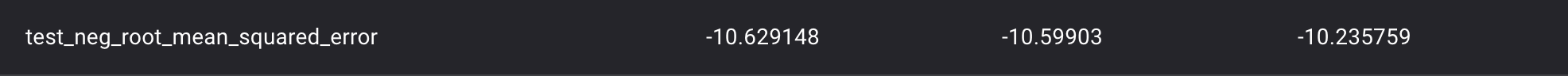The RMSE of the validation is the same, but the training RMSE value has decreased and is closer to the validation value.
This means that the degree of overfitting has decreased.

Another parameter that prevents the model from overfitting is feature_fraction, which adjusts the ratio of features randomly selected for each iteration.
Let's change the value of this feature_fraction to 0.8 and create a model.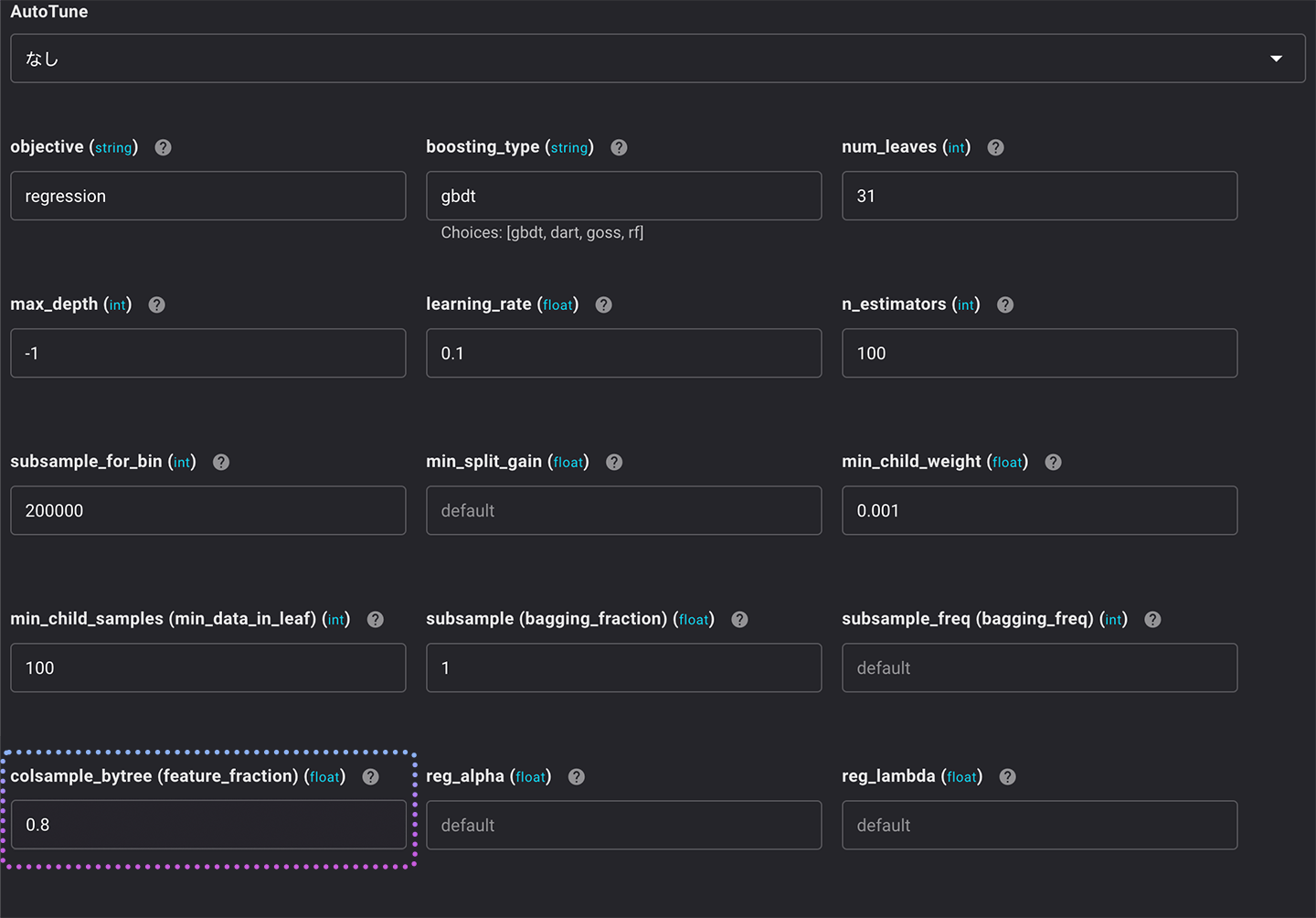If we set the parameters to this value, the model will use 80% of the features in each iteration.
The results are as follows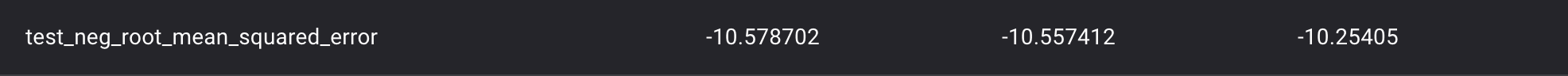The RMSE value of the training has been further improved.

The next step is to adjust the values of bagging_fraction and bagging_freq.
bagging_fraction allows us to randomly sample rows for each iteration. similar to feature_fraction, bagging_fraction is done on rows. bagging_freq adjusts the frequency of updating the sampled rows. Similar to feature_fraction, but bagging_fraction is done on rows. bagging_freq is a parameter that can be set to adjust the frequency at which the sampled rows are updated.
You can use the following parameters: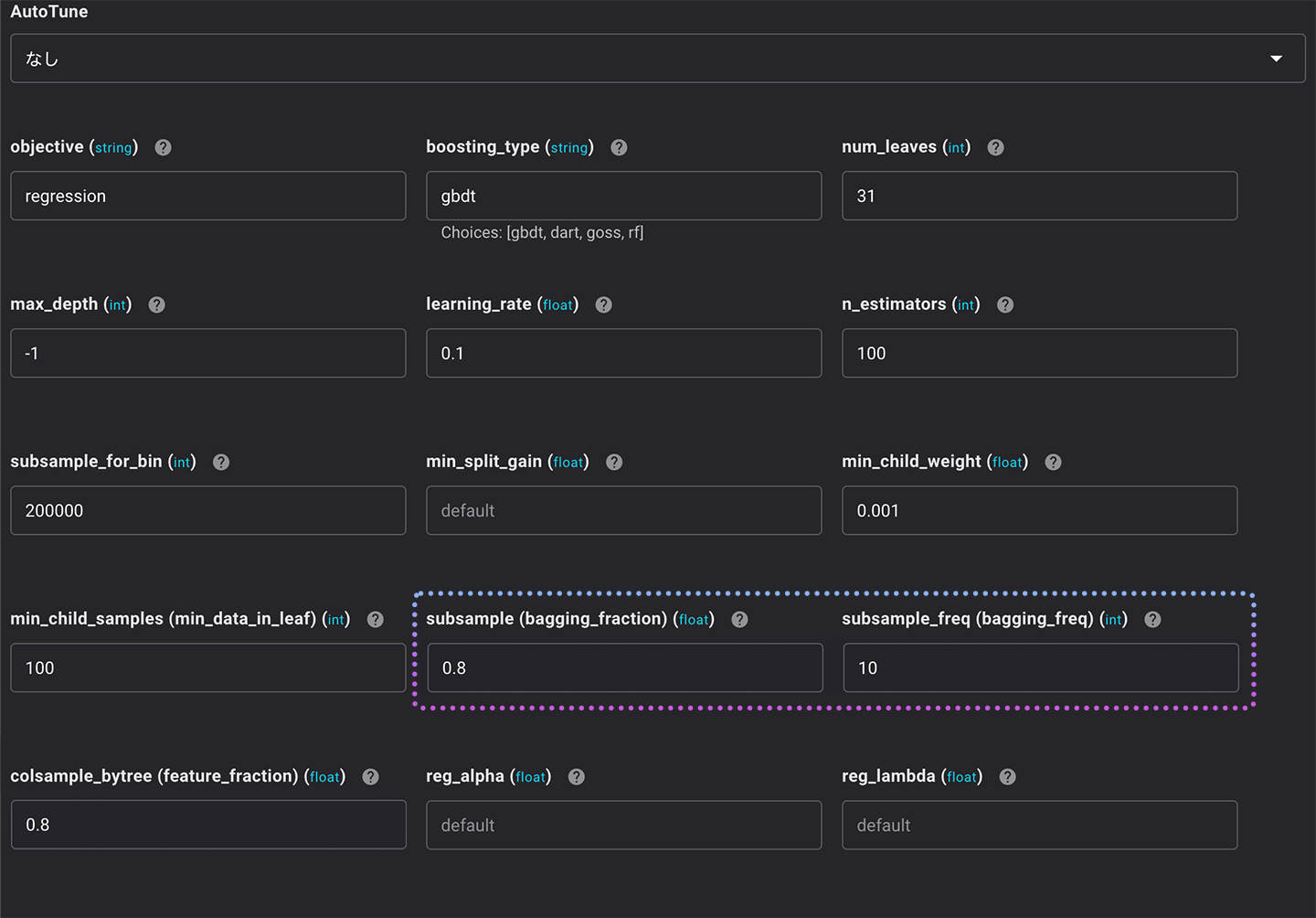• bagging_fraction: 0.8
• bagging_freq: 10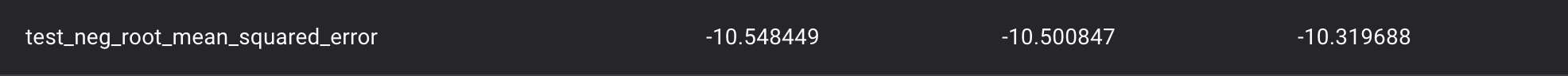The difference between the training RMSE and the validation RMSE has been further reduced.

The next parameters to be tuned are max_depth, num_leaves, and learning_late.
LightGBM is an algorithm that uses ensemble learning by combining decision trees with boosting techniques. Therefore, the complexity of individual trees can also be a cause of overfitting.
The complexity of a tree is adjusted by max_depth and num_leaves, where max_depth adjusts the maximum depth of the tree and num_leaves adjusts the maximum number of leaves the tree can have.

Since LightGBM adapts to the growth of the tree on a leaf-by-leaf basis, it is important to adjust the two parameters max_depth and num_leaves together.

Finally, there is learning_rate. In general, the smaller the learning_rate, the better the performance will be, but the slower the model will be trained, so it should be set considering the amount of data and machine specs.
In this case, we set it to 0.001 since we do not have a lot of data.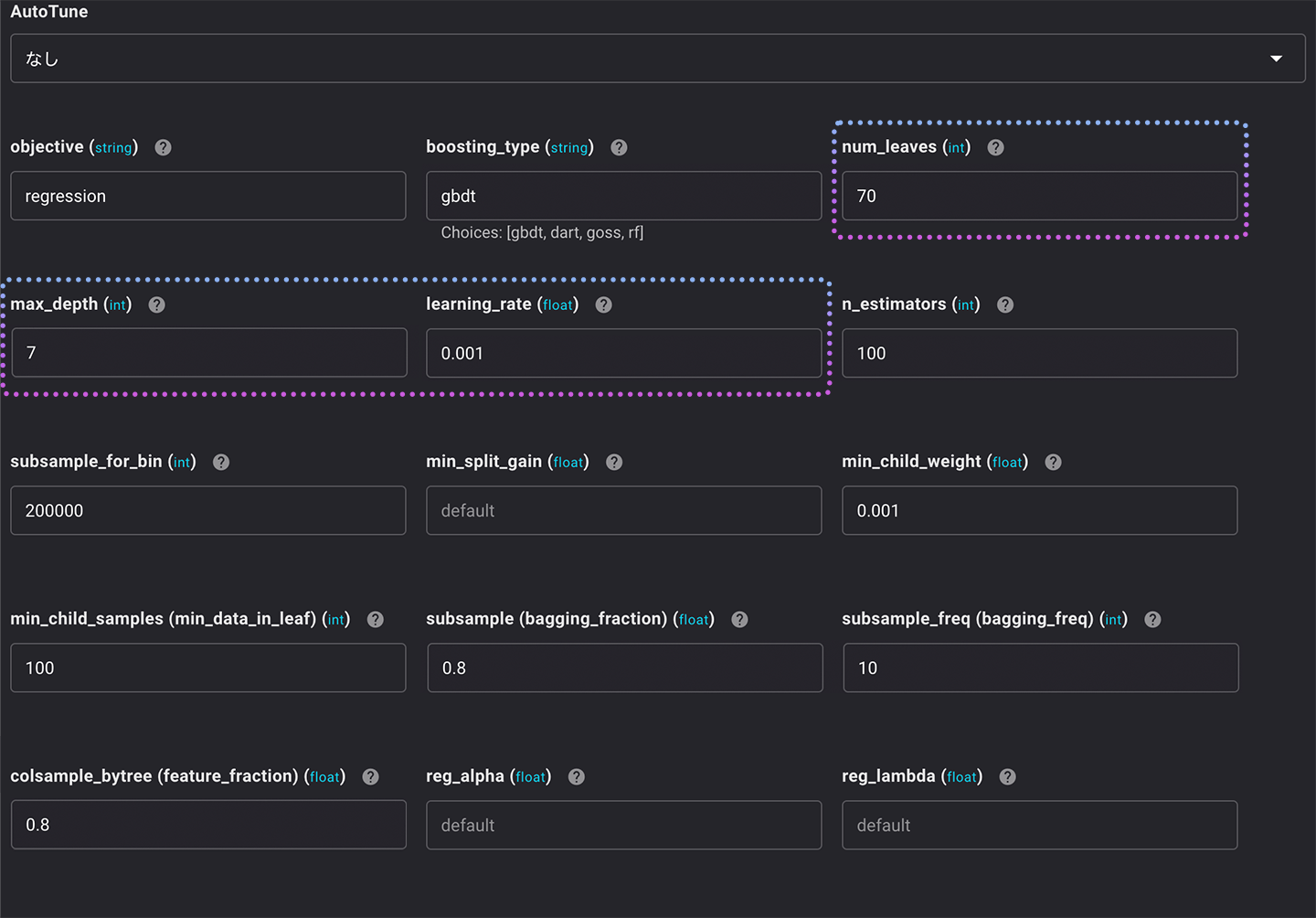• max_depth：7
• num_leaves：70
• learning_rate：0.001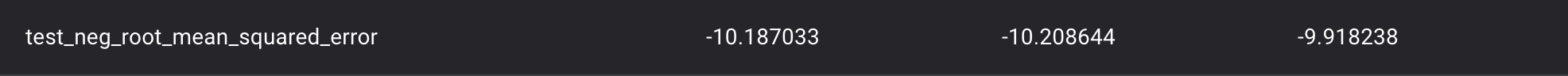The difference between the RMSE of training and the RMSE of validation decreased further.

Thus, by adjusting the hyperparameters, we can prevent the model from overtraining.
However, if the performance of the model in the first place does not reach the target value at all, there is a limit to what can be achieved by adjusting the hyperparameters.
In such a case, first review the quantity and quality of the data and perform feature engineering, etc., and then tune the hyperparameters when the model performance is close to the target value.

VARISTAは機械学習モデルの開発、管理をノーコードで効率的に行うことができる新しいプラットフォームです。
データをお持ちでしたらすぐに始められますので、是非ともご相談ください。
Stay in Touch